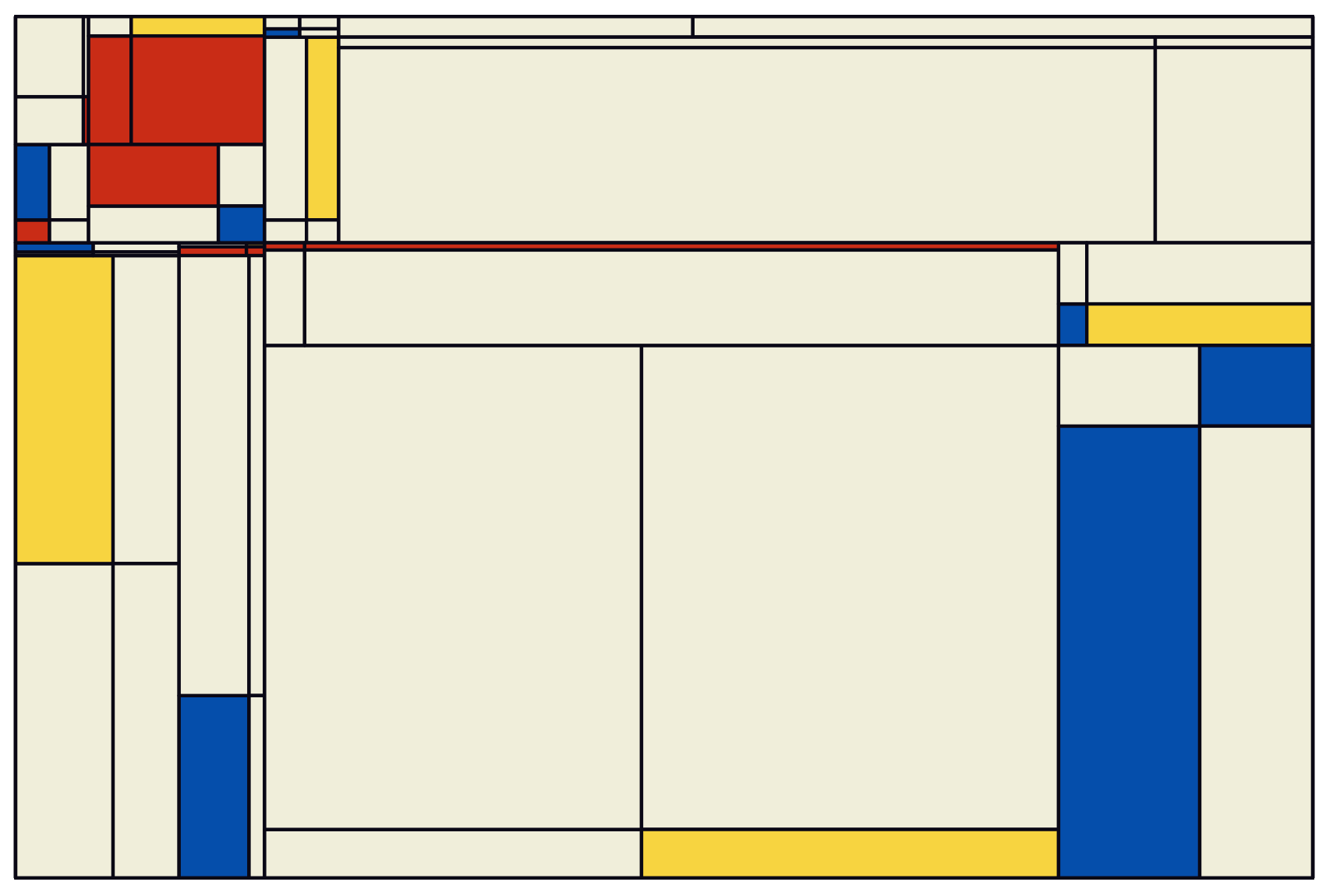## Quick use

library(mondrianaRt)
%>% <- magrittr::%>%
tileSet() %>% plotTiles()## Art customization

tileSet() %>% plotTiles()

The ratio of the plot is controlled by the ratio argument (1 by default, i.e. a square plot).

tileSet(ratio = 1/3) %>% plotTiles()

How “deep” the plot is (i.e. how recursive the internal loop is) is controlled by the levels argument (4 by default).

tileSet(levels = 10) %>% plotTiles()

By default, some tiles are randomly split in 2 (either vertically or horizontally), rather than in 4. This is controlled by the filter argument (TRUE by default).

tileSet(levels = 10, filter = FALSE) %>% plotTiles()

One can also control the minimum stretch (both horizontally and vertically) of each tile. By default, this ensures that the tiles are somewhat consistent. This can be reduced/increased with the min.stretch argument (0.04, or 4% by default)

tileSet(levels = 10, filter = FALSE, min.stretch = 0.01) %>% plotTiles()

This option can also be deactivated by using min.stretch = 0. This leads to a large increase of tiles, since they are all considered valid !

tileSet(levels = 10, filter = FALSE, min.stretch = 0) %>% plotTiles()

## Many plots at once

lapply(1:50, function(seed) {
message(seed)
set.seed(seed)
ratio <- sample(seq(0.5, 1.5, by = 0.1), 1)
level <- sample(2:8, 1)
filter <- sample(c(TRUE, FALSE))
whites <- sample(seq(0.2, 0.8, by = 0.1), 1)
stretch <- sample(seq(0.02, 0.10, 0.001))
p <- tileSet(
ratio = ratio,
levels = level,
seed = seed,
filter = filter,
min.stretch = stretch
) %>% plotTiles(white.density = whites)
ggplot2::ggsave(glue::glue('mondrian_seed-{seed}.png'), w = 10, h = 10*ratio)
})

## Acknowledgments

I got some inspiration from the previous attempts to reproduce/enhance the art style from Piet Mondrian: